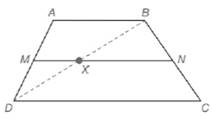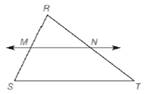Chapter 5.6, Problem 34EElementary Geometry For College St...

7th Edition
Alexander + 2 others
ISBN: 9781337614085

Solutions

Chapter
SectionElementary Geometry For College St...

7th Edition
Alexander + 2 others
ISBN: 9781337614085
Textbook Problem

Use Exercise 33 and the following drawing to complete the proof of this theorem: “The length of the median of a trapezoid is one-half the sum of the lengths of the two bases”. Given: Trapezoid A B C D with median M N ¯Prove: M N = 1 2 ( A B + C D ) 33. Use Theorem 5.6.1 and the drawing to complete the proof of this theorem: “If a line is parallel to one side of a triangle and passes through the midpoint of a second side, then it will pass through the midpoint of the third side.” Given: Δ R S T with M the midpoint of R S ; ¯ M N ↔ ∥ S T ¯Prove: N is the midpoint of R T ¯

To determine

To prove:

MN=12(AB+CD).

Explanation

Given:

We need to prove, “The length of the median of a trapezoid is one-half the sum of the lengths of the two bases”.

In a trapezoid ABCD, MN¯ is the median.

Property used:

Means-Extremes Property:

In a proportion, the product of the means equals the product of the extremes; that is, if ab=cd (where b0 and d0), then ad=bc.

If a line is parallel to one side of a triangle and intersects other two sides, then it divides these sides proportionally.

Proof:

Consider a trapezoid ABCD.

Given that MN¯ is the median.

We know that the median of the trapezoid is parallel to each base.

MN¯AB¯CD¯

We know that, if a line is parallel to one side of a triangle and passes through the midpoint of a second side, then it will pass through the midpoint of the third side

Still sussing out bartleby?

Check out a sample textbook solution.

See a sample solution

The Solution to Your Study Problems

Bartleby provides explanations to thousands of textbook problems written by our experts, many with advanced degrees!

Get Started

Convert the expressions in Exercises 8596 radical form. (x1/2y1/3)1/5

Finite Mathematics and Applied Calculus (MindTap Course List)

Evaluate the limit, if it exists. limx4x2+95x+4

Single Variable Calculus: Early Transcendentals

True or False: is a convergent series.

Study Guide for Stewart's Multivariable Calculus, 8th

For y = 3x + tan x, y = _____. a) 3 + sec x b) 3x sec2 x + 3 tan x c) 3 + sec2 x d) x cos x + sin x

Study Guide for Stewart's Single Variable Calculus: Early Transcendentals, 8th